# Ⅴ. Portfolio margin mode: cross margin trading

Published Mar 21, 2023Updated Aug 30, 202313 min read

Portfolio Margin mode allows users to trade spot, margin, perpetual swaps, futures, and options within one account and uses a risk-based model that determines margin requirements.

This model takes into consideration positions in spot, margin, perpetual swaps, futures and options combined, thus potentially reducing the margin requirements of a portfolio. Portfolio Margin allows for effective margin coverage while preserving efficient use of capital.

Similar to multi-currency margin mode, the user's equity in different currencies will be converted into an equivalent USD amount to be used as the margin for order placement and holding positions.

### How can I be qualified for Portfolio Margin?

To be qualified for portfolio margin, you:

*Must maintain minimum net equity of 10,000 USD.

**Must declare to have an understanding of the concept of portfolio margin.

### How does risk-offsetting work in Portfolio Margin?

OKX portfolio margin offers two modes:

1. Derivatives-only mode: All derivatives are grouped into risk units based on their underlyings (e.g. BTC-USD, BTC-USDT, ETH-USD, ETH-USDT, etc). The perpetual swaps, futures, and options with the same underlying are considered holistically within the risk unit. Margins are calculated per risk-unit, so as to factor in risk-offsetting among instruments.

Note: under OKX risk-unit groupings, crypto-margined and USDT-margined contracts are considered as different risk-units.

2. Spot-derivatives risk offset mode: Including spot into risk unit for margin calculation so that margin requirement could be significantly lowered if derivatives are hedged with spot.

Note: Under spot-derivatives risk offset mode, the user could choose to place spot in either USDT or crypto-margined risk unit.

*Spot-derivatives (USDT) risk offset mode: the underlying spot is placed into USDT-margined risk unit.

**Spot-derivatives (Crypto) risk offset mode: the underlying spot is placed into crypto-margined risk unit.

Example:

Mode SOL-USDT Risk Unit SOL-USD Risk Unit
Derivatives-only SOL-USDT Perpetual swaps, futures SOL-USD perpetual swaps, futures, options
Spot-derivatives (usdt) SOL-USDT Perpetual swaps, futures & SOL SOL-USD Perpetual swaps, futures, options
Spot-derivatives (crypto) SOL-USDT Perpetual swaps, futures SOL-USD Perpetual swaps, futures, options & SOL

When the user enters Portfolio Margin for the first time, the default mode will be spot-derivatives risk offset mode - USDT (2a).

To switch on/off spot-derivatives risk offset mode, or choose derivatives-type to be offsetted, user shall go to settings as below.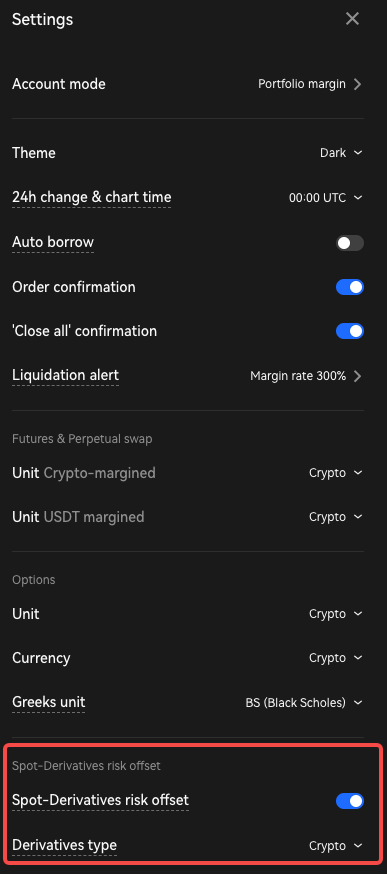User can see "Spot in use" in the "Position"&"Assets" at the bottom of trading page.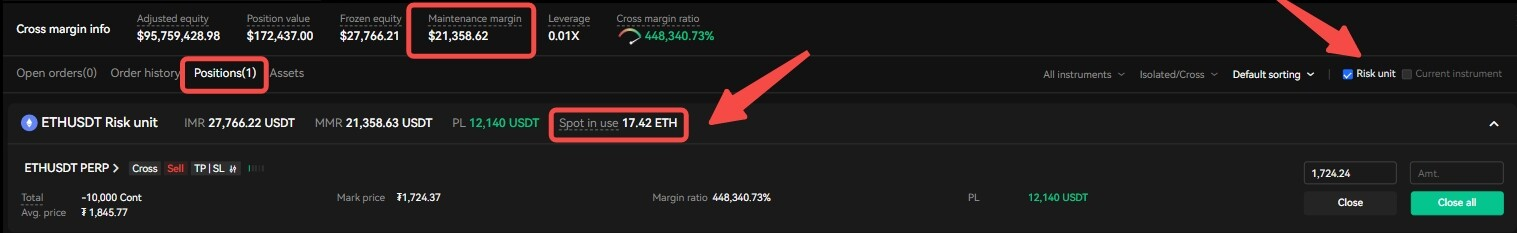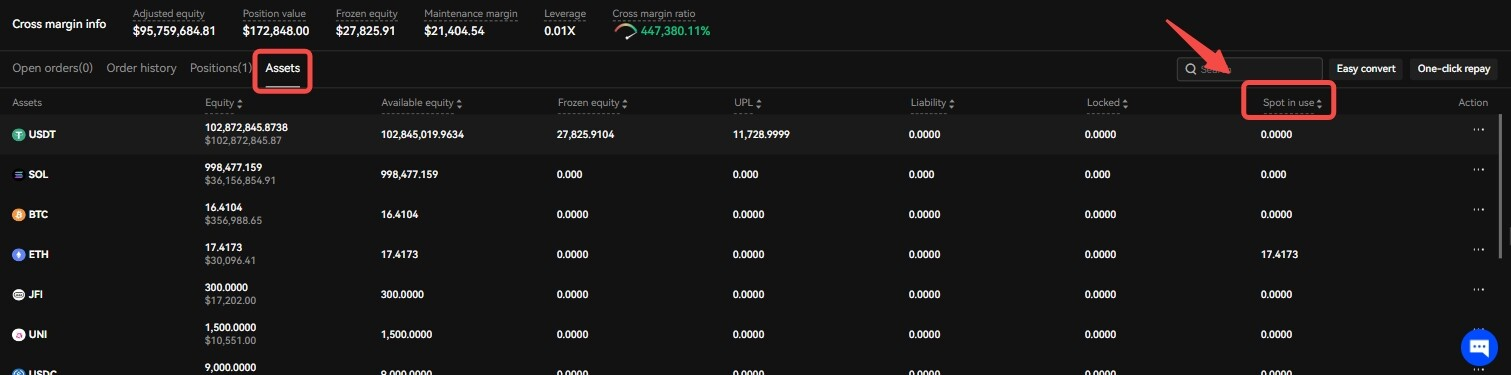### How does Portfolio Margin calculate?

Maintenance Margin Requirement (MMR) is determined in risk unit basis, where all instruments (perpetual swaps, futures, options, and spot under the spot-derivatives risk offset mode) are grouped by underlyings to simulate the maximum loss that can occur in a portfolio under a specific set of market conditions. The USD-value of the individual MMRs will then be summed up into a portfolio MMR (in USD value)

Initial Margin Requirement (IMR) is then derived from MMR.

Spot-derivatives risk offset mode: Spot in Use

Under spot-derivatives risk offset mode, spot in use, or how much spot is used under the risk unit is determined by the delta of derivatives that belong to the same risk unit. Spot is only added when derivatives of the corresponding risk unit can be hedged with the spot.

For each risk unit

1. If spot equity >0 & derivatives delta < 0, spot in use = min (|equity|, |derivatives delta|)

2. If spot equity <0 & derivatives delta > 0, spot in use (borrowing) = -min(|equity|, |derivatives delta|)

3. Otherwise, spot in use = 0.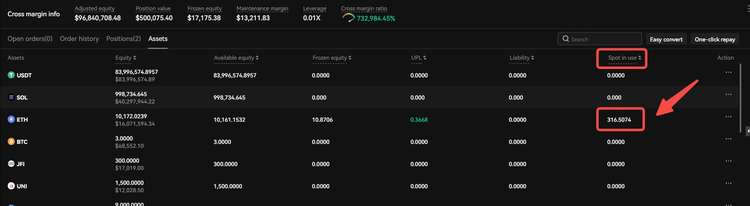Portfolio Margin Components

Portfolio margin consists of derivatives margin and borrowing margin. Portfolio margin calculates sums up derivative margin under each risk unit and then adds borrowing margin to obtain the margin at portfolio level.

MMR = Sum of USD value of each risk unit Derivatives MMR+ account level Borrowing MMR

IMR = 1.3 * Max (Positive-delta derivatives MMR, Negative-delta derivatives MMR, total derivatives MMR) + Borrowing IMR

Where,

Positive-delta derivative MMR = MR required for all derivative positions + MR required for all open derivative order with positive delta(+ MR required for all derivative orders in RFQ in Liquid Market Place)

Negative-delta derivative MMR = MR required for all derivative positions + MR required for all open derivative order with negative delta(+ MR required for all derivative orders in RFQ in Liquid Market Place)

Total derivatives MMR = MR required for all derivative positions(+ MR required for all derivative orders in RFQ in Liquid Market Place)

A. Derivatives MMR

Derivatives margin calculates 6 risks (MR1-6) by stress testing the portfolio under a specific set of market conditions of each risk unit, and then applying the minimum charge (MR7). Minimum charge is designed to cover any liquidation fee, transaction cost, and slippage. Details of corresponding market conditions are available in Appendix.

MR1: Spot shock (applicable for all derivatives / and spot under spot-derivatives risk offset mode)

MR2: Theta decay risk (applicable for options only)

MR3: Vega risk (applicable for options only)

MR4: Basis risk (applicable for all derivatives)

MR5: Interest rate risk (applicable for options only)

MR6: Extreme move (applicable for all derivatives / and spot under spot-derivatives risk offset mode)

MR7: Adjusted minimum Charge (applicable for all derivatives)

Derivatives MMR = max { [max(spot shock, theta decay risk, extreme move) + basis risk + vega risk + interest rate risk], adjusted minimum charge}

B. MR8: Borrowing MMR/IMR

Borrowing margin calculation calculates margin based on the borrowing amount on each underlying per corresponding tiers. Please refer to margin ratio defined here.

Both auto-borrow mode and non-borrow mode are available. In auto-borrow mode, the user could sell a currency unavailable in account through spot or derivatives when the total USD-equivalent equity at portfolio level is sufficient. Borrowings could include margin borrowing, options buyer borrowing, and negative UPL (unrealized profit and loss) caused by opening derivatives positions as well as borrowing used for opening derivatives positions.

Please note MR8 also include the borrowings that might be required to cover derivatives MMR.

C. Margin Ratio Calculation

*The USD value of margin required:

MMR/IMR of different currencies under each risk unit are converted into an equivalent USD value by spot indices.

**The USD value of the portfolio equity

Due to the differences in market liquidity of each currency, the platform calculates the equivalent USD value of each currency in the portfolio equity based on certain discount rates. Please refer the latest discount rate table.

***The margin ratio of the portfolio is then determined by USD value of the portfolio equity divided by USD value of margin required.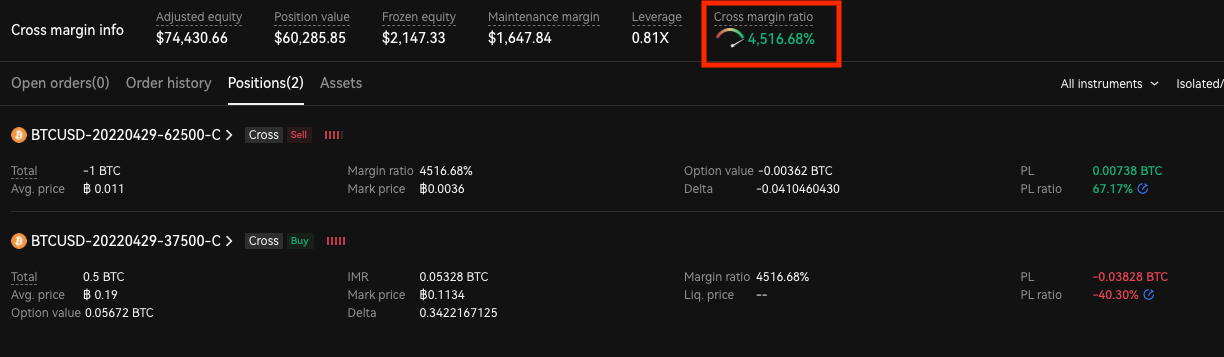### How can I test portfolio margin on existing/simulated portfolio?

Position Builder and corresponding API is available for the user to test the portfolio margin for the existing positions (even if the user is using other margin modes), as well as any simulated portfolio.

1. The user can simulate new positions in position builder. IMR and MMR are shown as below. The user can also include the existing positions with the simulated positions, such that he/she can also simulate the impact on IMR and MMR.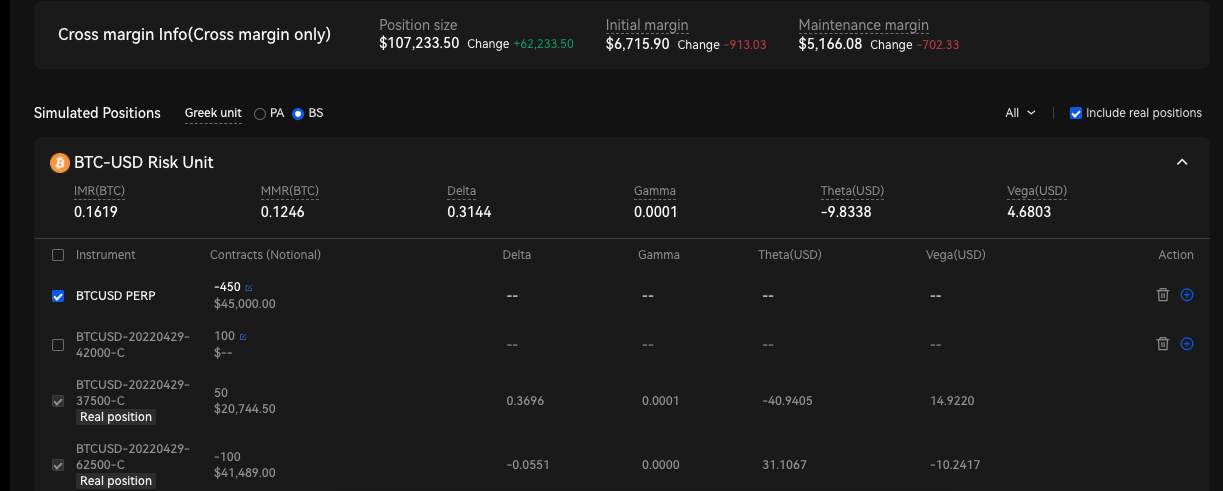2. When the mouse is hovering around MMR, the system will show the breakdown of MMR based on the above MR scenarios.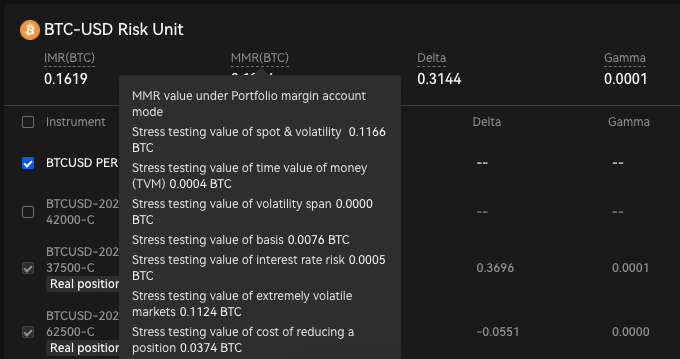### What will happen during liquidation?

When the margin ratio is lower than a certain threshold (eg. 300%), the system will send a liquidation alert to the account owner.

The liquidation in portfolio margin mode is triggered based on whether the margin ratio reaches 100%. Due to potentially low liquidity of options, liquidation could result in new perpetual swaps or futures positions being opened, however, only to decrease the risk profile of the whole portfolio.

The liquidation process will execute sequential steps. The flow will go to the next step if the account is still at risk after the previous one is completed and can never be undone. The flow is completed when the account backs to a safe status ( margin ratio >120%) or all the positions in the account are liquidated.

#### Step 1. Dynamic hedging process (DDH)

DDH refers to the use of the DDH principle to reduce the overall risk of the portfolio margin account by adjusting the positions of perpetual swaps/future. DDH is applicable on option positions when spot shock risk (MR1) is largest among all risks (eg. BTCUSD index down 15%)

#### Step 2. Basis hedge process

Basis hedge process is to reduce the basis risk by liquidating futures at different expiry dates at the same time when basis risk (MR4) is the largest among all risks. (basis risk means the risk of different contract prices for the same underlying with different expiry dates).

#### Step 3. General position reducing process

General position reducing process prioritizes the positions that can make the account positions safer to reduce the account maintenance margin. The number of positions to be reduced is determined by both default system configurations (the liquidity of each instrument) and the tradable positions.

### Appendix: Margin Calculations

Please note all the parameters listed below are subject to change based on market environment.

MR1: Spot shock

Spot shock scenario is comprised of market simulations moving the underlying up or down or unchanged in combination with moving implied volatility up or down or unchanged. MR1 is the maximum loss in these 21 market simulations (7 price movement simulations * 3 implied volatility change simulations; Implied volatility change could be in volatility points or percentage; MR1 takes the maximum loss)

Price Move

Underlying Currency Maximum Price Move
BTC, ETH +/- 0%, 5%, 10%, 15%
LTC, BCH, EOS, OKB, DOT, BSV, LINK, FIL, ADA, TRX, UNI, XRP +/- 0%, 7%, 14%, 20%
Others +/- 0, 8%, 16%, 25%

Implied Volatility Change (Option only) in points or in %

Time to Expiry Maximum Implied Volatility Change in Points Maximum Implied Volatility % Change
0 days 30 (e.g IV = 50%, after shock IV = 80% or 20%) 50 (e.g IV = 50%, after shock IV = 75% or 25%)
30 days 25 35
60 days 20 25

#### MR2: Time decay risk (option only)

Time decay risk measures 24 hour decay in portfolio value assuming no change in underlying price move and implied volatility.

#### MR3: Vega term structure risk (option only)

Vega term structure risk arises from differences in option value for the same underlying with different expiry dates under same implied volatility shock. It measures the risk of change in implied volatility across different expiry dates that is not captured in MR1.

#### MR4: Basis risk

Basis risk arises from differences in contract prices for the same underlying with different expiry dates. The futures with further away expiry dates are likely to be more volatile than the futures that are closer to expiry. It measures the risk of change in forward price across different expiry dates.

Basis risk scenario is comprised of different market simulation, moving the forward price or forward basis up on positive delta on each expiry.

Underlying Currency Maximum Forward Basis move Maximum Forward Price Move
BTC, ETH 30%* 1.5%*
LTC, BCH, EOS, OKB, DOT, BSV, LINK, FIL, ADA, TRX, UNI, XRP 35% 2%
Others 40% 2.5%

*Example:

Forward price at expiry 1 is 3000 USD/ETH

Forward price at expiry 2 is 3500 USD/ETH

Spot is 3200 USD/ETH

Simulated maximum forward price basis move at expiry 1 is -200 *30% and maximum forward price move at expiry is 3000 * 1.5%

Simulated maximum forward price basis move at expiry 2 is 300 *30% and maximum forward price move at expiry is 3500 * 1.5%

#### MR5: Interest rate risk (option only)

Interest rate risk measures the risk of change in yield curve by populating a principal component analysis (PCA) style rate movement table. Interest rate here refers to interest rate used in option pricing model.

PC1 explains parallel shift in yield curve. PC2 explains the slope change of yield curve.

Days 1 7 14 30 90 180 360 720
PC1 3 2 1.75 1.5 1 0.9 0.8 0.7
PC2 4 3 2 1 0 -0.5 -0.75 -0.9

Interest rate risk scenario is comprised of 8 market simulations by moving the yield curve proportionally to PC1 and PC2. For instance, scenarios include moving the existing yield curve by +/- 5% * PC1, +/- 2.5% * PC1, +/- 3% * PC2, +/- 2% * PC1. MR5 is the maximum loss that occurs in these 8 market simulations.

#### MR6: Extreme move

Extreme move scenario is similar to spot shock scenario but the maximum underlying price move doubles the largest spot shock in MR1 and implied volatility remains unchanged. Extreme move is designed to capture the scenarios where options might be exercised when the underlying experiences extreme move. MR6 takes half of the maximum loss under 2 scenarios. For instance, MR6 of BTC-USD risk unit is half of the maximum loss when BTC-USD moves + 30% or -30%, whichever is larger. If the risk unit only contains perpetual swaps and futures, MR6 = MR1.

Underlying Currency Maximum Price Move
BTC, ETH +/- 30%
LTC, BCH, EOS, OKB, DOT, BSV, LINK, FIL, ADA, TRX, UNI, XRP +/- 40%
Others +/- 50%

#### MR7: Minimum charge

Step 1. Raw minimum charge

Raw minimum charge is designed to cover any slippage, and transaction cost.

Raw minimum charge per contract = slippage per contract + transaction cost per contract

Futures/Perpetual swaps minimum charge

Transaction cost per contract = taker fee * contract value * contract multiplier

Slippage per contract depends on the first tier futures MMR in unified account.

Options minimum charge

Transaction cost per contract = min (taker fee * contract value * contract multiplier, 12.5% * mark price * contract value * contract multiplier)

For long positions: slippage per contract = min{max(min_per_delta, min_per_delta *abs(delta)), Mark Price} * contract_multiplier

For short positions, slippage per contact = max(min_per_delta, min_per_delta * abs(delta)) * contract_multiplier

Take BTC as an example, minimum charge per delta (min_per_delta) =0.02

Adjusted Minimum charge is derived from a scaling factor function based on futures/perpetual swaps and option short raw minimum charge under each risk unit. Scaling factor is designed to reflect the slippage to liquidate portfolios with different sizes.

Adjusted minimum charge MR7 = (Futures + perpetual swaps + option short_Raw minimum charge)*Scaling factor + option long_raw minimum charge

Tier configuration on BTC, ETH

Tier (i) Range of the raw minimum charge (in USD) Multiplier
1 [0 ~7,000] 1
2 (7,000 ~16,000] 2
3 (16,000 ~29,000] 3
4 (29,000 ~43,000] 4
5 (43,000 ~69,000] 5
6 (69,000 ~95,000] 6
7 (95,000 ~121,000] 7
8 (121,000 ~147,000] 8
9 (147,000 ~ +∞) 9

Tier configuration on other underlying

Tier (i) Range of the raw minimum charge (in USD) Multiplier
1 [0 ~3,000] 1
2 (3,000 ~8,000] 2
3 (8,000 ~14,000] 3
4 (14,000 ~19,000] 4
5 (19,000 ~27,000] 5
6 (27,000 ~36,000] 6
7 (36,000 ~45,000] 7
8 (45,000~54,000] 8
9 (54,000 ~63,000] 9
10 (63,000 ~72,000] 10
11 (72,000 ~81,000] 11
12 (81,000 ~90,000] 12
13 (90,000 ~+∞) 13

#### MR8: Borrowing Margin

In auto-borrow mode, the user could sell a currency unavailable in account through spot or derivatives when the total USD-equivalent equity is sufficient. A potential borrowing amount will be generated in the user's account and some of the adjusted equity will be used as margin requirement. When the currency's equity is less than 0 due to overselling or the derivative loss is settled in the currency, the real liability and the corresponding interest of this currency will be generated automatically.

In non-borrow mode, the user could only use the available balance or available equity of the currency to place orders of spot, futures, perpetual swap,and options. There may be a situation in which the equity of a certain currency cannot pay off the loss settled in this currency. In this situation, if the account has surplus equity in other currencies and the overall USD value of the account is sufficient, the account will be safe, and the real liability will be passively generated. If the liability is within the interest-free range of the currency, no interest will be charged. When the liability of the currency exceeds the interest-free limit, a forced repayment (TWAP) will be triggered. The system will automatically use available equity of other currencies in the account to cover the liability and make it stay within the interest-free limit. TWAP will be executed by selling the positive assets into USDT and repaying the liability with USDT.

Potential borrowing = actual borrowing + virtual borrowing

Actual borrowing refers to margin borrowing, Options buyer borrowing, and negative UPL (unrealized profit and loss) caused by opening positions. Actual borrowing occupies the user's Margin tier limit, main account limit, and the platform's total lending limit.

Virtual borrowing refers to borrowing used for opening positions. The virtual borrowing occupies the user's Margin tier limit, but does not occupy the user's main account limit and the platform's total lending limit.

The negative UPL caused by the opening positions in actual borrowing enjoys the interest-free limit. While the borrowing caused by Margin borrowing, Options buyer borrowing, and the realized profit and loss of the contract does not enjoy the interest-free limit.

Please refer to interest calculation for the details of the interest-free limit rules.